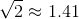Scan QR code or get instant email to install app

Question:

# Which is bigger: the cube root of 54 or the square root of 18?

A Square root of 18
explanation

1212In this type of problem it will be easier to approximate than to solve for the actual roots of both numbers. Factor down the numbers by using prime factorization and making use of as many repeated small numbers.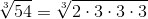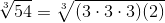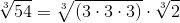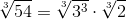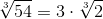While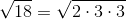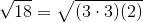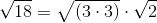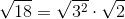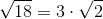Compare the two values: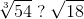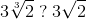Equivalently, ask the question: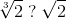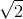is bigger than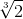; so work your way back up.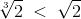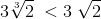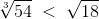TIP: How do you know that:is bigger than? Quite simple:had to be multiplied by itself 3 times to get 2, whilehad to be multiplied by itself 2 times only to get 2. Using the calculator: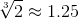, while